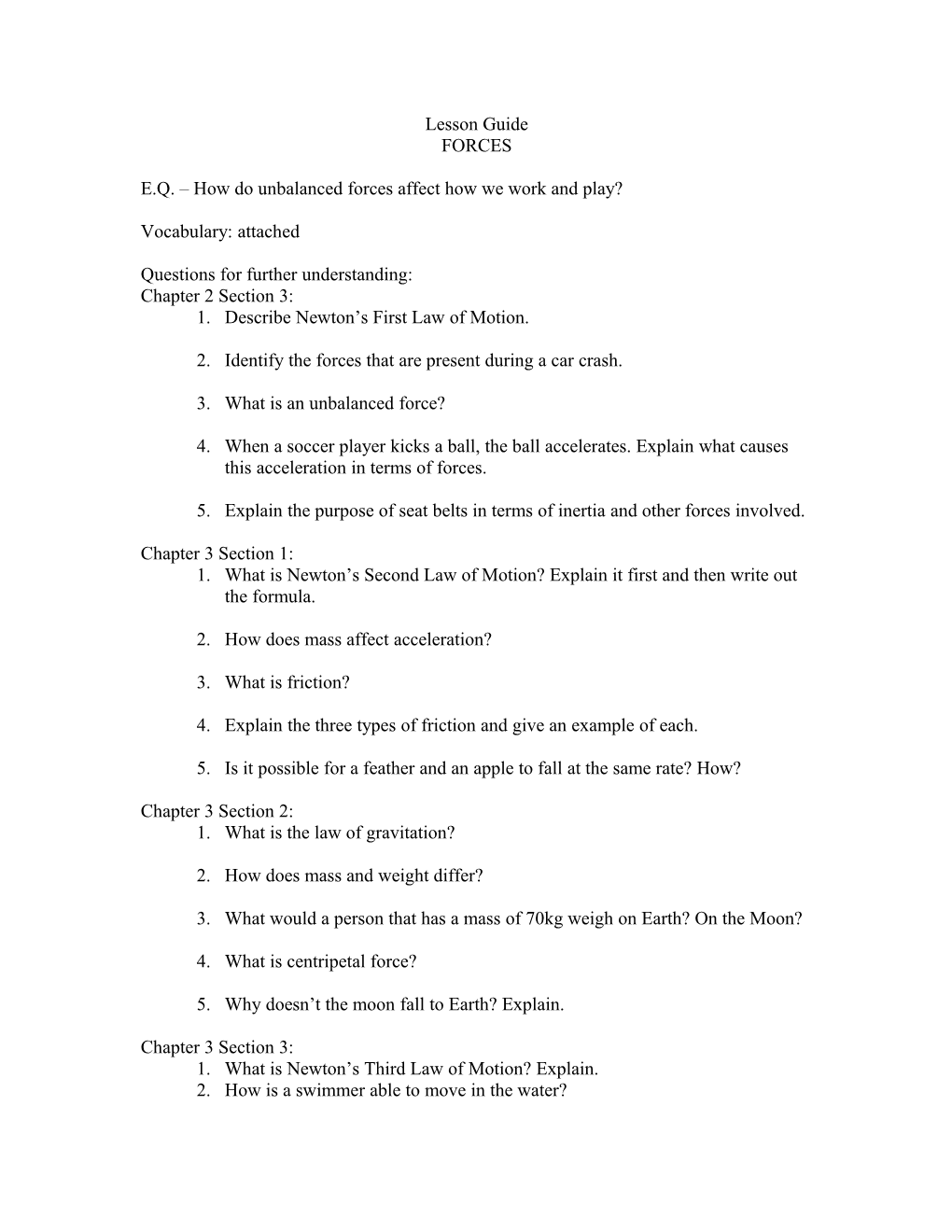# E.Q. How Do Unbalanced Forces Affect How We Work and Play?Lesson Guide

FORCES

E.Q. – How do unbalanced forces affect how we work and play?

Vocabulary: attached

Questions for further understanding:

Chapter 2 Section 3:

1. Describe Newton’s First Law of Motion.
1. Identify the forces that are present during a car crash.
1. What is an unbalanced force?
1. When a soccer player kicks a ball, the ball accelerates. Explain what causes this acceleration in terms of forces.
1. Explain the purpose of seat belts in terms of inertia and other forces involved.

Chapter 3 Section1:

1. What is Newton’s Second Law of Motion? Explain it first and then write out the formula.
1. How does mass affect acceleration?
1. What is friction?
1. Explain the three types of friction and give an example of each.
1. Is it possible for a feather and an apple to fall at the same rate? How?

Chapter 3 Section 2:

1. What is the law of gravitation?
1. How does mass and weight differ?
1. What would a person that has a mass of 70kg weigh on Earth? On the Moon?
1. What is centripetal force?
1. Why doesn’t the moon fall to Earth? Explain.

Chapter 3 Section 3:

1. What is Newton’s Third Law of Motion? Explain.
2. How is a swimmer able to move in the water?
1. How can a rocket move through outer space where no matter exists for it to push against?
1. Explain the Law of Conservation of Momentum.
1. When two pool balls collide, what happens to the momentum of each?

PRACTICE PROBLEMS:

1. The diagram below shows the position of a cross-country skier at various times. At each of the indicated times, the skier turns around and reverses the direction of travel. In other words, the skier moves from A to B to C to D. Use the diagram to determine the distance traveled by the skier and the resulting displacement during these three minutes. Then depress the mouse on the pop-up menu below to see the answer.2. While on vacation, Lisa Carr traveled a total distance of 440 miles. Her trip took 8 hours. What was her average speed?

3. Seymour Butz views football games from under the bleachers. He frequently paces back and forth to get the best view. The diagram below shows several of Seymour's positions and times. At each marked position, Seymour makes a "U-turn" and moves in the opposite direction. In other words, Seymour moves from position A to B to C to D. What is Seymour's average speed and average velocity? Depress the mouse on the pop-up menu below to see the answer.